Function Repository Resource:

# TrendLine

Trend line of a given set of data

Contributed by: Jan Mangaldan
 ResourceFunction["TrendLine"][{{x1,y1},{x2,y2},…}] returns an InfiniteLine representing the least-squares line fit of the points {xi,yi}.

## Examples

### Basic Examples (3)

Here is some data:

 In:=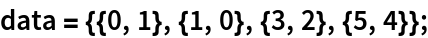The trend line for the data:

 In:=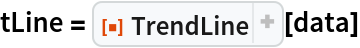Out=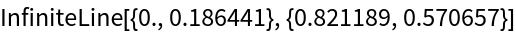Show the data and the trend line together:

 In:=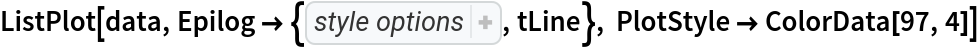Out=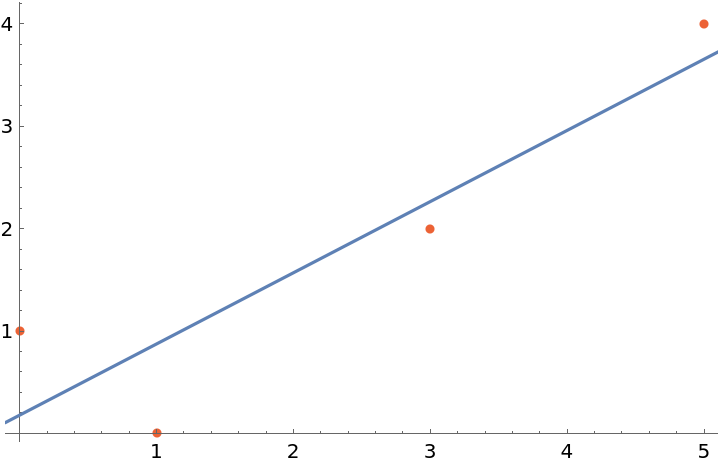### Properties and Relations (3)

Here is some data:

 In:=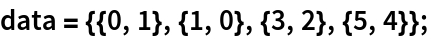The linear function corresponding to the result of TrendLine is equivalent to the results returned by functions such as Fit and LinearModelFit:

 In:=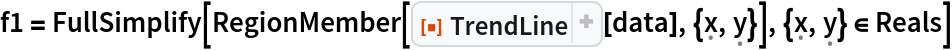Out=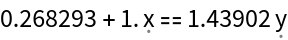In:=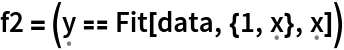Out=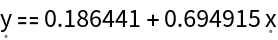In:=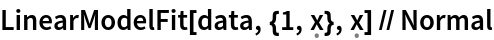Out=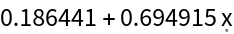Show the lines together:

 In:=Out=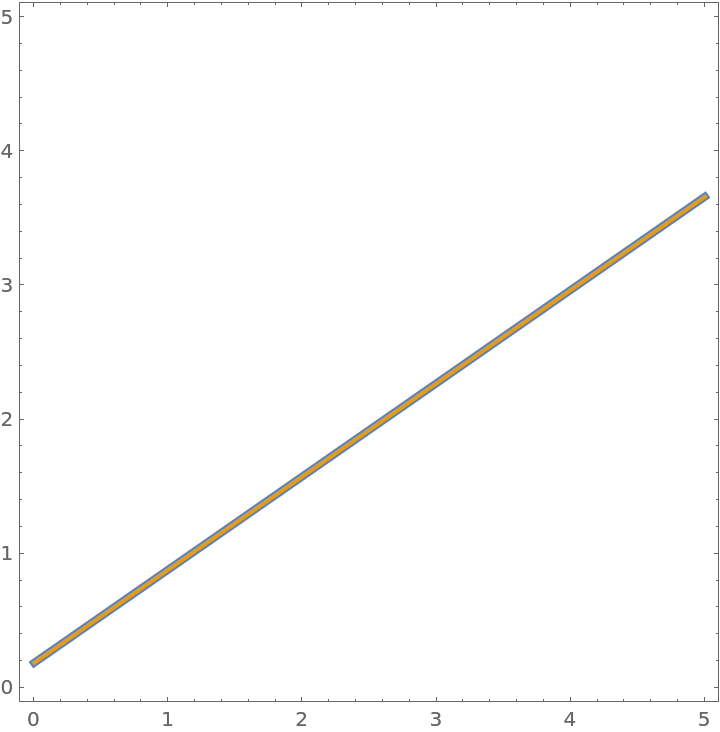## Version History

• 1.0.0 – 02 August 2022

## Author Notes

Trend lines corresponding to other fitting norms (e.g. 1, ∞) will be implemented in a future version.